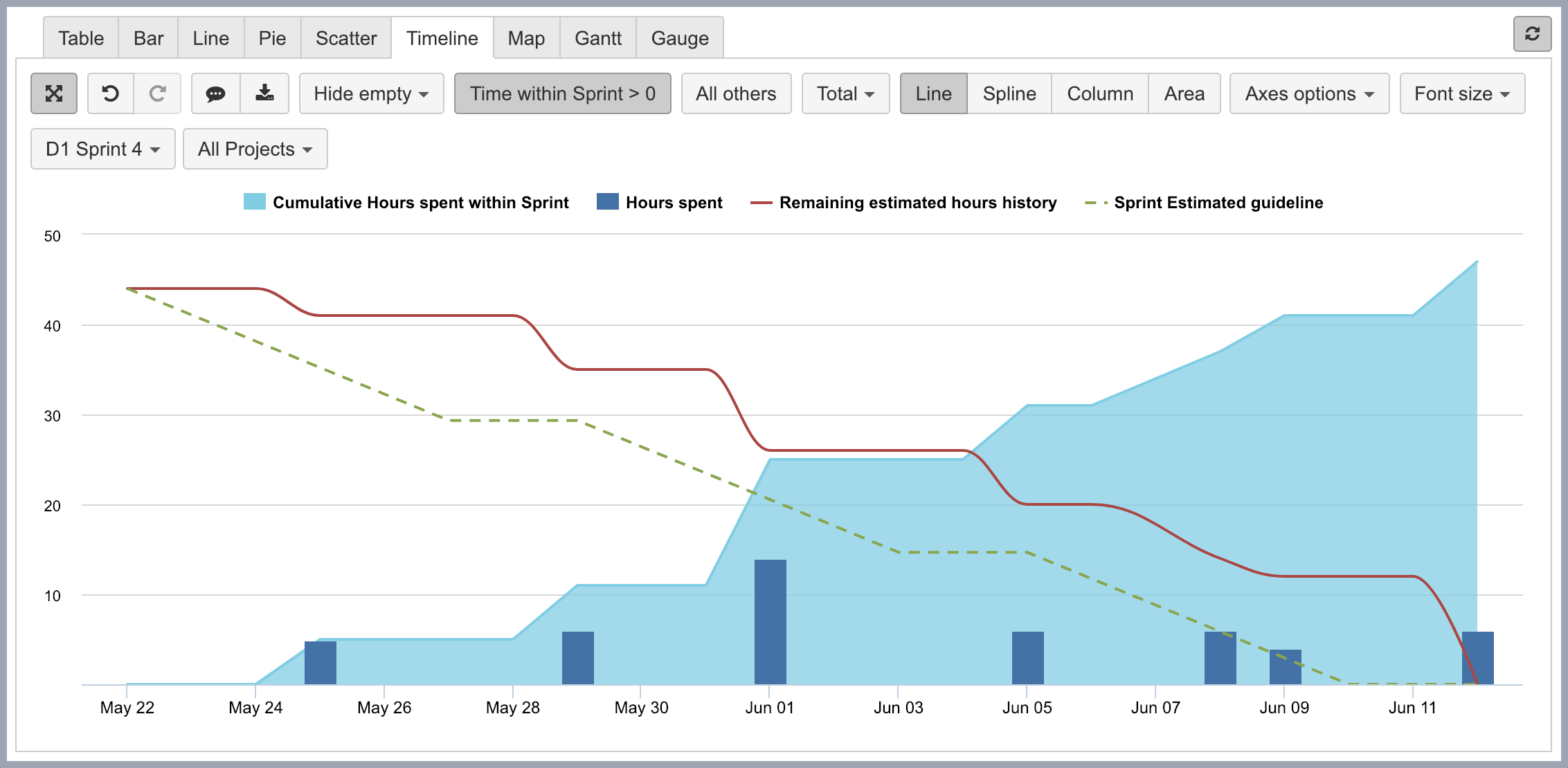All eazyBI for Jira eazyBI for Confluence Private eazyBI
All eazyBI for Jira eazyBI for Confluence Private eazyBI

# Time tracking burndown in selected Sprint eazyBI for Jira

Below you can see an example of time tracking burndown in for selected Sprint. This report is available also in eazyBI Demo account where you can get report definition:

This report shows the guideline of how many hours should be spent per each day depending on the estimate in the beginning of Sprint; logged hours as well as how many hours are remaining after hours have been logged. Remaining estimate history displays changes if, for example, new task with new estimate is added to Sprint when the Sprint has already started.This report uses Measures dimension on columns. Before creating report you should select import of Remaining estimated hours history calculated measure. When imported, you should be able to select this measure from Calculated members / Default. Select measure Hours spent as well for the report.

The other two Measures are calculated with following formulas (Note that these measures will show any results only when Sprint is selected, you should add it to Pages):

• Cumulative Hours spent within Sprint which sums up Hours spent starting from first Sprint day until current Time member.

```CoalesceEmpty(Sum(
Filter(
[Time].CurrentHierarchyMember.Level.Members,
[Measures].[Time within Sprint] = 1
).Item(0):[Time].CurrentHierarchyMember,
[Measures].[Hours spent]
), 0)```
• Sprint Estimated guideline which calculates suggested amount of hours that should be spent per each day of Sprint by multiplying Remaining estimated hours in the beginning of Sprint with working days left until end of Sprint and dividing that with how many working days are in Sprint.

```-- annotations.group = Time tracking
CASE WHEN
DateBetween([Time].CurrentHierarchyMember.StartDate,
DateWithoutTime([Sprint].CurrentMember.get('Start date')),
[Sprint].CurrentMember.get('End date') ) OR
DateInPeriod(
[Sprint].CurrentMember.get('Start date'),
[Time].CurrentHierarchyMember )
THEN
[Measures].[Sprint Remaining Estimates committed] *
(
DateDiffWorkdays(
DateWithoutTime([Sprint].CurrentMember.get('Start date')),
DateWithoutTime([Sprint].CurrentMember.get('End date'))
) -
DateDiffWorkdays(
DateWithoutTime([Sprint].CurrentMember.get('Start date')),
[Time].CurrentHierarchyMember.StartDate)
) /
DateDiffWorkdays(
DateWithoutTime([Sprint].CurrentMember.get('Start date')),
DateWithoutTime([Sprint].CurrentMember.get('End date')) )
END```

On rows there is Time dimension with Day level selected from All hierarchy level members. To display only days that are within Sprint, select Time within Sprint calculated measure from Measures dimension Calculated members / Other properties (this measure should be added when importing Sprint custom field as dimension).

Put Sprint dimension on pages and select particular sprint you are interested in. Now Time within Sprint should show 1 for those dates which are within selected sprint start and end dates.

Click on Time within Sprint column header and select Filter rows and add condition > 0 (after that you should see just days within sprint).

Now you can click on Time within Sprint column header and select Remove – column will be removed but condition will remain.